# Algebra II : Sigma Notation

## Example Questions

### Example Question #21 : Sigma Notation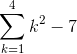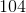Explanation:

This notation is asking us to add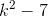for all integer values of k between 1 and 4: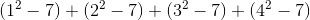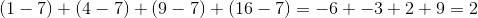### Example Question #22 : Sigma Notation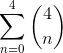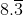Explanation:

This expression is asking us to add the expressions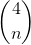for every integer value of n from 0 to 4: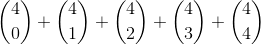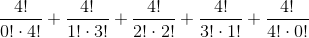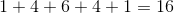### Example Question #23 : Sigma Notation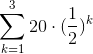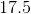Explanation:means that we are adding half of 20, plus half of that, plus half of that.

1: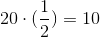2: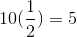3: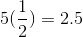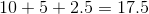### Example Question #24 : Sigma Notation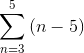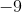Explanation:

This is asking us to add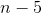for n = 3 and 4.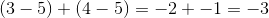### Example Question #25 : Sigma Notation

Calculate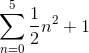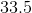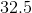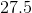Explanation:

This is asking us to plug in the integers between 0 and 5, then add these numbers together.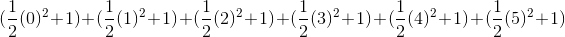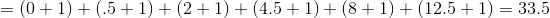### Example Question #26 : Sigma Notation

Evaluate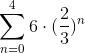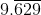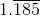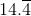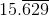Explanation:

This is asking us to add 6 plus two thirds of 6, plus two thirds of that, etc.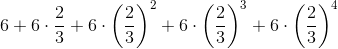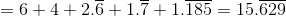### Example Question #27 : Sigma Notation

Evaluate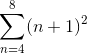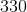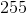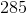Explanation:

This is asking us to substitute integer values between 4 and 8 for n, and then add the results.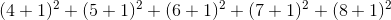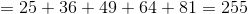### Example Question #28 : Sigma Notation

Evaluate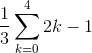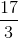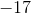Explanation:

First, evaluate the sigma expression. It is asking us to plug in for k all of the integers between 0 and 4: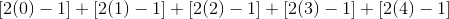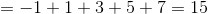Now, multiply by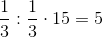### Example Question #29 : Sigma Notation

Solve: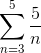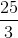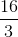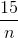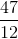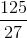Explanation:

Substitute the terms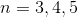for each iteration of the summation.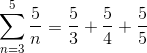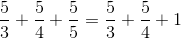Convert the fractions to a common denominator.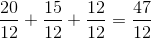The answer is:### Example Question #30 : Sigma Notation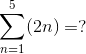Explanation:

When using summation notation, the number on the bottom is the starting input value, and the number on the top is the ending input value.This means that you will be adding the result of the first 5 outputs after plugging in 1 through 5.

Plug in each value of n, from 1 to 5.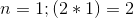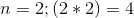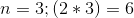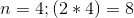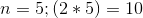Now we take all of these numbers and sum them to get: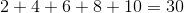so.....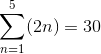### All Algebra II Resources